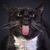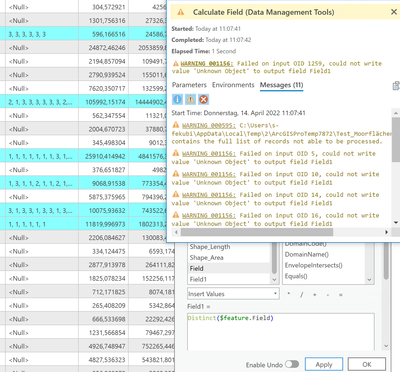# How to: Delete identical text after joining values

342
9
04-13-2022 05:37 AM
Labels (3)by
New Contributor II

Hey Community,

after spatial join, is there a way to summarize the values in the text field for each row? E.g. from "1,1,2,2,3,1,2" to: "1,2,3"?

Thanks!

-Felix1 Solution

Accepted SolutionsbyMVP Esteemed Contributor

You can sort the array as numbers with this addition

``````if (!IsEmpty(\$feature.Field)){
var array = Split(\$feature.Field, ',');
var array1 = [];
for (var k in array) {
Push(array1, Number(array[k]));
}
return Concatenate(Distinct(Sort(array1)), ',')
}``````
9 RepliesOccasional Contributor III

I think this should work if you use a python function (instead of print you'll want to use return.... I didn't have ArcPro available to test it so I used the python playground at W3Schools).  A little more python could sort the ouput:

``````test = "1,2,2,1,3,3,3,3,4,5,1,5,10"
test2 = []
test3 = ""
z = 1
for x in test.split(","):
test2.append(x)
myset = set(test2)
for y in myset:
if z == 1:
test3 = y
else:
test3 = test3+","+y
z = z + 1
print(test3)``````byMVP Esteemed Contributor

In Arcade, the Distinct function returns the unique values of an array

``````Distinct([1,1,2,1,1,2,2,3,4,5])
// Returns [1,2,3,4,5]``````by
New Contributor II

Thanks! Normally it should work... .

But the Field Calculator cannot calculate any result. Both fields have the same format (Text). Any idea what I'm doing wrong?byMVP Esteemed Contributor

Since your input is a string, you first have to turn it into an array using the Split function. Then you can use the Distinct function. Finally, you have to turn that array back into a text string using the Concatenate function.

This example also adds a test so that it ignores any Null strings. If that test is left off, the calculated field will contain an empty string instead of a Null.

``````if (!IsEmpty(\$feature.Field)){
var array = Split(\$feature.Field, ',');
var array1 =  Distinct(array);
return Concatenate(array1, ',')
}``````Occasional Contributor III

@KenBuja That return Concatenate made me think python MUST have something similar and sure enough... thank you for that:

``````test = "1,2,2,1,3,3,3,3,4,5,1,5,10"
test2 = []
for x in test.split(","):
test2.append(x)
myset = sorted(set(test2),key=int)
test = ','.join(myset)
print(test)

#returns  1,2,3,4,5,10
#String on numbers sorted as numbers ``````by
New Contributor II

Ah, okay. Works perfect! Thank you. And when I want to sort these arrays, do I have to write the Sort.function before Return.function? (e.g: return Concatenate(Sort(array1, ',')))

When I write it in the same line, there is an error called "Not callable value".byMVP Esteemed Contributor

Great! Don't forget to click the "Accept as Solution" buttonOccasional Contributor III

The ',' is a parameter of the concatenate function, but the way you have it above your passing it to sort and sort doesn't know what to do with it. Try

return concatenate(Sort(array1),',')

This will sort your array values as strings (i.e. '1,10,11,2,3,33,4').byMVP Esteemed Contributor

You can sort the array as numbers with this addition

``````if (!IsEmpty(\$feature.Field)){
var array = Split(\$feature.Field, ',');
var array1 = [];
for (var k in array) {
Push(array1, Number(array[k]));
}
return Concatenate(Distinct(Sort(array1)), ',')
}``````